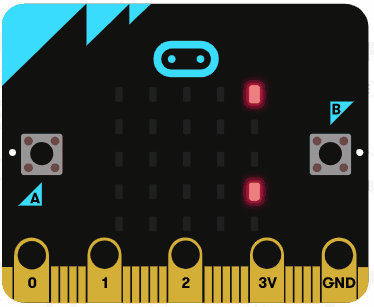JavaScript
Hard
60 mins
445 points
What you need:
• Computer/laptop

# JavaScript Operators

In this lesson we learn about JavaScript Operators, what they are and how we use them in JavaScript.

### 1 - What is an Operator?

If we look at the following sum: 7 + 4 = 11

The + is the operator and the 7 and the 4 are the operands.

So the numbers are called operands and the operation (to be performed between the two operands) is defined by an operator.

In JavaScript we can use the following types of operators.

Type Description
Arithmetic Operators
For adding, subtracting, multiplication and division.
String Operators
For joining strings together.
Assignment Operators
For assigning values to variables.
Comparison Operators
For comparing two different values (equal to, greater than, less than etc.)
Logical Operators
For determining the logic between variables or values (and, or, not).

### 2 - Arithmetic Operators

Arithmetic operators perform arithmetic on numbers. The main arithmetic operators are:

Operator DescriptionExample
+
For adding two numbers together.
``let answer = 4 + 5``
-
For subtracting one number from another.
``let answer = 6 - 3``
*
For multiplying two numbers together.
``let answer = 2 * 7``
/
For dividing one number by another.
``let answer = 10 / 2``

There are also some other arithmetic operators that we can use:

Operator DescriptionExample
++
For incrementing a number (increasing it by 1).
``answer++``
--
For decrementing a number (decreasing it by 1).
``answer--``
%
Modulus operator (%) returns the division remainder.
``let answer = 8 % 3``

### 3 - Try it out

Let's try using the arithmetic operators. Go to the https://makecode.microbit.org website, create a new project and switch from Blocks to JavaScript.

Add the following code examples one by one to your project and see if you get the same result in the simulator.

Add this code Result
``````let answer = 4 + 5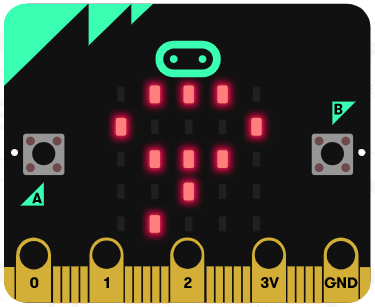``````let answer = 6 - 3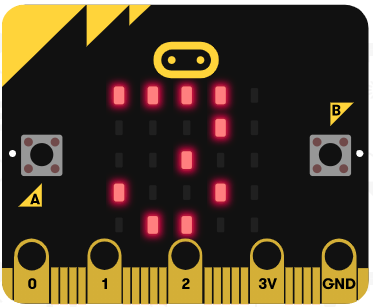``````let answer = 2 * 7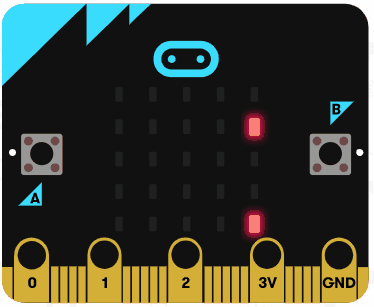``````let answer = 10 / 2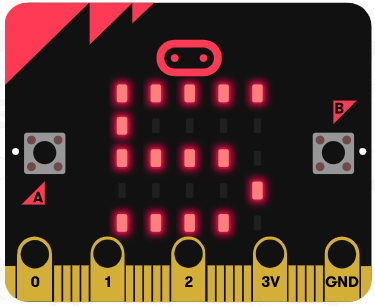``````let answer = 5

basic.forever(function () {
})

input.onButtonPressed(Button.A, function () {
})``````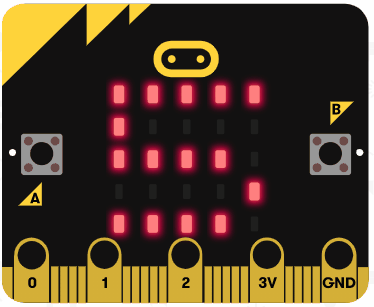``````let answer = 5

basic.forever(function () {
})

input.onButtonPressed(Button.A, function () {
})

// add this code
input.onButtonPressed(Button.B, function () {
})``````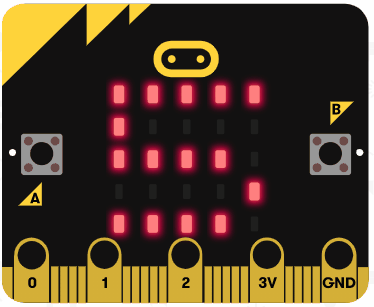### 4 - String Operators

The + operator can also be used to join (concatenate) strings together.

``````let firstName = "Stefan"
let lastName = "Jones"
let fullName = firstName + " " + lastName
``````

This will make fullName equal to Stefan Jones.

If you add strings and numbers together then JavaScript will treat the number as a string.

``````let fname = "Jane"
let age = 25
let x = fname + age
``````

This will make x equal to Jane25.

### 5 - Try it out

Let's try using the string operators. Delete all the previous code that you added.

Add the following code examples one by one to your project and see if you get the same result in the simulator.

Add this code Result
``````let firstName = "Stefan"    // put in your own name
let lastName = "Jones"      // put in your own name
let fullName = firstName + " " + lastName
basic.showString(fullName)``````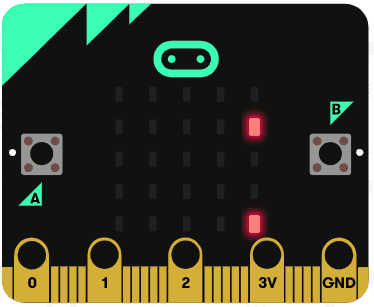``````let fname = "Jane"  // put in your own name
let age = 25        // put in your own age
let x = fname + age
basic.showString(x)``````